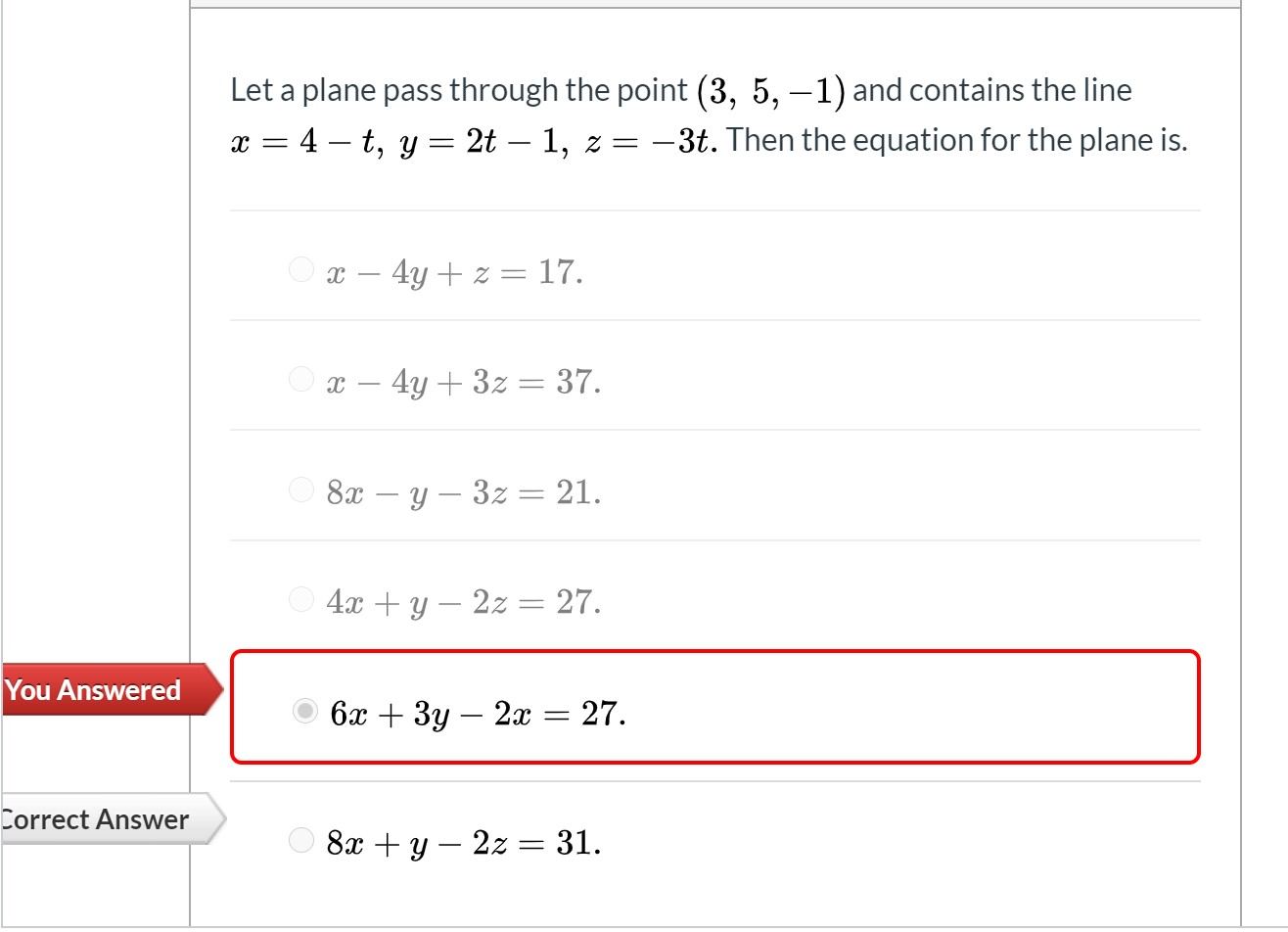# Let a plane pass through the point (3, 5, –1) and contains the line x = 4 – t, y = 2t – 1, z = -3t. Then the equation for the plane is. Ox – 4y + z = 17. Ox – 4y + 3z= 37. O 8x – y – 3z = 21. O 4x + y – 2z= 27. бх + Зу — 2а 3D27. 8х + у — 2z — 31.

Questionhelp_outlineImage TranscriptioncloseLet a plane pass through the point (3, 5, –1) and contains the line x = 4 – t, y = 2t – 1, z = -3t. Then the equation for the plane is. Ox – 4y + z = 17. Ox – 4y + 3z= 37. O 8x – y – 3z = 21. O 4x + y – 2z= 27. бх + Зу — 2а 3D27. 8х + у — 2z — 31. fullscreen

1 Rating

### Want to see this answer and more?

Experts are waiting 24/7 to provide step-by-step solutions in as fast as 30 minutes!*

*Response times may vary by subject and question complexity. Median response time is 34 minutes for paid subscribers and may be longer for promotional offers.
Tagged in
MathCalculus

### Other